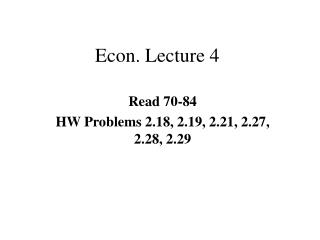DownloadDownload PresentationEcon. Lecture 4

# Econ. Lecture 4

Download Presentation## Econ. Lecture 4

- - - - - - - - - - - - - - - - - - - - - - - - - - - E N D - - - - - - - - - - - - - - - - - - - - - - - - - - -
##### Presentation Transcript

1. Econ. Lecture 4 Read 70-84 HW Problems 2.18, 2.19, 2.21, 2.27, 2.28, 2.29

2. Finishing interest formula’s (Uniform payment, Linear and Geometric Gradients)We finished with:

3. Solving this equation for A: Known as the Uniform Sinking Fund Factor, (A/F, i, n) Finding A, given F, i, n

4. Since F = P(1+i)n, we can substitute this for F in the previous equation The Uniform Series Capital Recovery Factor, (A/P, i, n)

5. Solving this equation for P, The Uniform Series Present Worth Factor, (P/A, i, n)

6. All of these equations can be solved using interest tables, Appendix C. • Note, if the table doesn’t exist for a given interest rate, you have to use the equations.

7. Example: • \$100 deposited at the end of each year in savings account, 6%, for five years, what do you have? • A = \$100 • F = ? • n = 5 years • i = 6% • F = A(F/A,i,n) = 100(F/A,6%,5) = 100(5.637) \$563.70

8. Or Notice that the tables can make life a lot easier.

9. Recall on the first day, \$100 a month for 40 years (work from 25-65) at an 8% rate. • Bond = 8%, Stock Index Fund = 12% • F=? • A = \$1200 /year • i = 8%

10. F = \$1200(F/A,40,8%) = 1200 (259.0565) = \$310,867.80 • F = \$1200(F/A,40,12%) = 1200(767.0914) = \$920509.68 • Just for kicks, what if at age 20 you decided to save \$100/month • F = \$1200(F/A,45,12%) = 1200(1358.23) = \$1,629,876

11. Example: • You wish to make uniform deposits every three months to have \$10,000 at the end of 10 years. At 6% annual, how much would you have to deposit?  • F = \$10,000 • A = ? • n = 40 quarterly deposits • i= 1.5% per quarter • A = F(A/F,i,n) = F(A/F,1.5%,40) = \$10,000(0.184) = \$184

12. Example: • Buying a car. Total price is \$6200. The down payment is \$1240, with 48 monthly payments, with 1% per month. Payments are due at the end of each month. What is the monthly payment?

13. P = 6200 – 1240 = \$4960 • A = ? • n = 48 monthly payments • i = 1% • A = P(A/P,i,n) = 4960(A/P,1%,48) = 4960(0.0263) = \$130.45

14. Example: • Selling a house. Mortgage of \$232.50 for 10 years. Selling mortgage to a bank with 1% interest per month. How much will the bank pay for mortgage. • A = \$232.50 • n = 120 months • i = 1% • P = ?  • P = A(P/A,i,n) = \$232.50(P/A,1%,120) = 232.509*(69.701) = \$16,205.48

16. Sometimes cash flow will increase by a constant amount. 100 125 150 175 200 n=5 P

17. This can be broken into two components: 100 100 100 100 100 0 G 2G 3G (n-1) G + P* = P’

18. G = \$25, n = 5 • We can compute P by adding P’ with P*. We have an equation for P’, • P* = G(P/G,i,n)

19. Gradient series present worth factor, (P/G,i,N)

20. Example: • Maintenance on a machine is expected to be \$155 after one year and increase \$35 each year for seven years. How much should be set aside now for the upcoming eight years. i = 6% • P = 155(P/A,6%,8) + 35(P/G,6%,8) = 155(6.210) + 35(19.841) = \$1656.99